# 0.8 Exponential functions and graphs

 Page 1 / 2

## Introduction

In Grade 10, you studied graphs of many different forms. In this chapter, you will learn a little more about the graphs of exponential functions.

## Functions of the form $y=a{b}^{\left(x+p\right)}+q$ For $b>0$

This form of the exponential function is slightly more complex than the form studied in Grade 10.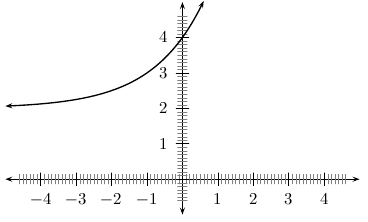General shape and position of the graph of a function of the form f ( x ) = a b ( x + p ) + q .

## Investigation : functions of the form $y=a{b}^{\left(x+p\right)}+q$

1. On the same set of axes, with $-5\le x\le 3$ and $-35\le y\le 35$ , plot the following graphs:
1. $f\left(x\right)=-2·{2}^{\left(x+1\right)}+1$
2. $g\left(x\right)=-1·{2}^{\left(x+1\right)}+1$
3. $h\left(x\right)=0·{2}^{\left(x+1\right)}+1$
4. $j\left(x\right)=1·{2}^{\left(x+1\right)}+1$
5. $k\left(x\right)=2·{2}^{\left(x+1\right)}+1$
Use your results to understand what happens when you change the value of $a$ . You should find that the value of $a$ affects whether the graph curves upwards ( $a>0$ ) or curves downwards ( $a<0$ ). You should also find that a larger value of $a$ (when $a$ is positive) stretches the graph upwards. However, when $a$ is negative, a lower value of $a$ (such as -2 instead of -1) stretches the graph downwards. Finally, note that when $a=0$ the graph is simply a horizontal line. This is why we set $a\ne 0$ in the original definition of these functions.
2. On the same set of axes, with $-3\le x\le 3$ and $-5\le y\le 20$ , plot the following graphs:
1. $f\left(x\right)=1·{2}^{\left(x+1\right)}-2$
2. $g\left(x\right)=1·{2}^{\left(x+1\right)}-1$
3. $h\left(x\right)=1·{2}^{\left(x+1\right)}+0$
4. $j\left(x\right)=1·{2}^{\left(x+1\right)}+1$
5. $k\left(x\right)=1·{2}^{\left(x+1\right)}+2$
Use your results to understand what happens when you change the value of $q$ . You should find that when $q$ is increased, the whole graph is translated (moved) upwards. When $q$ is decreased (poosibly even made negative), the graph is translated downwards.
3. On the same set of axes, with $-5\le x\le 3$ and $-35\le y\le 35$ , plot the following graphs:
1. $f\left(x\right)=-2·{2}^{\left(x+1\right)}+1$
2. $g\left(x\right)=-1·{2}^{\left(x+1\right)}+1$
3. $h\left(x\right)=0·{2}^{\left(x+1\right)}+1$
4. $j\left(x\right)=1·{2}^{\left(x+1\right)}+1$
5. $k\left(x\right)=2·{2}^{\left(x+1\right)}+1$
Use your results to understand what happens when you change the value of $a$ . You should find that the value of $a$ affects whether the graph curves upwards ( $a>0$ ) or curves downwards ( $a<0$ ). You should also find that a larger value of $a$ (when $a$ is positive) stretches the graph upwards. However, when $a$ is negative, a lower value of $a$ (such as -2 instead of -1) stretches the graph downwards. Finally, note that when $a=0$ the graph is simply a horizontal line. This is why we set $a\ne 0$ in the original definition of these functions.
4. Following the general method of the above activities, choose your own values of $a$ and $q$ to plot 5 graphs of $y=a{b}^{\left(x+p\right)}+q$ on the same set of axes (choose your own limits for $x$ and $y$ carefully). Make sure that you use the same values of $a$ , $b$ and $q$ for each graph, and different values of $p$ . Use your results to understand the effect of changing the value of $p$ .

These different properties are summarised in [link] .

 $p<0$ $p>0$ $a>0$ $a<0$ $a>0$ $a<0$ $q>0$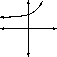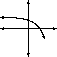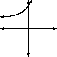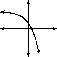$q<0$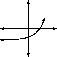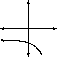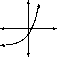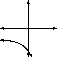## Domain and range

For $y=a{b}^{\left(x+p\right)}+q$ , the function is defined for all real values of $x$ . Therefore, the domain is $\left\{x:x\in \mathbb{R}\right\}$ .

The range of $y=a{b}^{\left(x+p\right)}+q$ is dependent on the sign of $a$ .

If $a>0$ then:

$\begin{array}{ccc}\hfill {b}^{\left(x+p\right)}& >& 0\hfill \\ \hfill a·{b}^{\left(x+p\right)}& >& 0\hfill \\ \hfill a·{b}^{\left(x+p\right)}+q& >& q\hfill \\ \hfill f\left(x\right)& >& q\hfill \end{array}$

Therefore, if $a>0$ , then the range is $\left\{f\left(x\right):f\left(x\right)\in \left[q,\infty \right)\right\}$ . In other words $f\left(x\right)$ can be any real number greater than $q$ .

If $a<0$ then:

$\begin{array}{ccc}\hfill {b}^{\left(x+p\right)}& >& 0\hfill \\ \hfill a·{b}^{\left(x+p\right)}& <& 0\hfill \\ \hfill a·{b}^{\left(x+p\right)}+q& <& q\hfill \\ \hfill f\left(x\right)& <& q\hfill \end{array}$

ok let me know some of the questions please.
Effah
ok am not wit some if den nw buh by tommorow I shall get Dem
Hi guys can I get Adam Smith's WEALTH OF NATIONS fo sale?
Ukpen
Wat d meaning of management
disaster management cycle
cooperate social responsibility
igwe
Fedric Wilson Taylor also define management as the act of knowing what to do and seeing that it is done in the best and cheapest way
OLANIYI
difference between microeconomics and macroeconomic
microeconomics is the study of individual units, firm and government while macroeconomics is the study of the economic aggregates.
okhiria
The classical theory of full employment
Lovely
what is monopoli power
the situation that prevails when economic forces balance so that economic variables neither increase nor decrease
Bombey
what is equilibrium
Kabir
what are the important of economic to accounting students with references
Economics is important because it helps people understand how a variety of factors work with and against each other to control how resources such as labor and capital get used, and how inflation, supply, demand, interest rates and other factors determine how much you pay for goods and services.
explain the steps taken by the government in developing rural market?
contribution of Adam smith in economics
I will join
Dexter
I will join
Patrick
Hey
Fatima
Hey
Amir
Hello
AS
hey
Umarou
I love this book and i need extra Economic book
Amir
Hey
Amir
what's happening here
AS
I love this book and i need extra Economic book
Amir
what is the meaning of function in economics
Pls, I need more explanation on price Elasticity of Supply
Is the degree to the degree of responsiveness of a change in quantity supplied of goods to a change in price
Afran
what is production
Humaira
Okay what is land mobile and land unmobile
scor
And what are the resources in land
scor
the proces of using the services of labor and equipmnt together with other in puts to make goods and services availble
Bombey
Okay what is land mobile and land unmobile
scor
Discuss the short-term and long-term balance positions of the firm in the monopoly market?
hey
Soumya
hi
Mitiku
how are you?
Mitiku
can you tell how can i economics honurs(BSC) in reputed college?
Soumya
through hard study and performing well than expected from you
Mitiku
what should i prepare for it?
Soumya
prepare first, in psychologically as well as potentially to sacrifice what's expected from you, when I say this I mean that you have to be ready, for every thing and to accept failure as a good and you need to change them to potential for achievement of ur goals
Mitiku
parna kya hai behencho?
Soumya
Hallo
Rabindranath
Hello, dear what's up?
Mitiku
cool
Momoh
good morning
Isaac
pls, is anyone here from Ghana?
Isaac
Afran
Afran
Hello
OLANIYI
pls anyone from Nigeria
OLANIYI
am a new candidate here, can someone put me 2ru
OLANIYI
hello
OLANIYI
Pls economic A level exam tomorrow pls help me
akinwale
am from Ghana
Jacob
Pls economic A level exam tomorrow pls help me
akinwale
Hi
Dev
bol Diya discuss ab krega v
Dev
hello Mr. Rabindranath
Dev
what do you want Dimlare
Dev
yes tell me your desire to have it
Dev
to have what?
OLANIYI
Good luck
JOSEPH
I want to know about economic A level tomorrow pls help
Lerato
okay
Umarou
okay
Umarou
hi
Humaira
hi
Liaqat
what is firms
A firm is a business entity which engages in the production of goods and aimed at making profit.
What is autarky in Economics.
what is choice
So how is the perfect competition different from others
what is choice
Tia
1
Naziru
2
Mayor
While the American heart association suggests that meditation might be used in conjunction with more traditional treatments as a way to manage hypertension
Researchers demonstrated that the hippocampus functions in memory processing by creating lesions in the hippocampi of rats, which resulted in ________.
The formulation of new memories is sometimes called ________, and the process of bringing up old memories is called ________.
Other chapter Q/A we can askByByBy